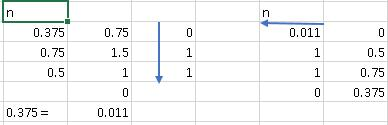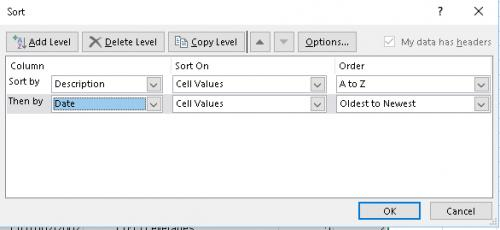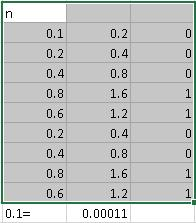Please Note: This article is written for users of the following Microsoft Excel versions: 2007, 2010, 2013, 2016, 2019, and Excel in Microsoft 365. If you are using an earlier version (Excel 2003 or earlier), this tip may not work for you. For a version of this tip written specifically for earlier versions of Excel, click here: Rounding in Results.

# Rounding in Resultsby Allen Wyatt
(last updated December 1, 2018)

Melissa was having a hard time understanding why Excel displays certain numbers in certain ways. She provided, as an example, the following steps:

2. In cell B2, enter 33.33333333.
3. In cell B3, enter 33.333333333 (one more digit than in step 2).
4. In cell C2, enter =B2*3.
5. In cell C3, enter =B3*3.

When Melissa would do this, cell C2 would display the result as 99.99999999, and cell C3 would display the result as 100. (You may have to widen column C to see these results.)

To see why Excel does this, there are a few things you have to understand. First of all, when you create a new worksheet, all the cells are formatted using the General format. Using this format, information is displayed in a general manner (thus the format name). In this format, numbers are displayed using up to ten digits. It doesn't matter whether the digits are to the left or right of the decimal place; only a maximum of ten are displayed.

What happens if the number that you are entering has more than ten digits, or if the result of a formula has more than ten digits? Then Excel rounds the number so that no more than ten digits are displayed. Thus, 99.99999999 is displayed, but if you add one more digit, the rounded result is 100. Rest assured, however, that Excel continues to maintain the cell contents with fifteen digits of accuracy, even though fewer digits are displayed.

If the number you are entering contains nothing to the right of the decimal point, but there are more than ten digits in the number, then the General format results in the number being displayed using scientific notation.

There is one caveat to the above: if the General-formatted cell into which the value is placed is narrower than what is required to display the full number, then the value is rounded so that it can be displayed within the available column width. If you later adjust the column width so it is wider, then the number is reformatted so it is displayed with up to ten digits.

If you want to use more than ten digits to display your number, you need to explicitly format the cell. Either click on the Increase Decimal tool (Number group, Home tab of the ribbon) or display Format Cells dialog box and use the controls on the Number tab to control how the displayed number should be formatted.

ExcelTips is your source for cost-effective Microsoft Excel training. This tip (8032) applies to Microsoft Excel 2007, 2010, 2013, 2016, 2019, and Excel in Microsoft 365. You can find a version of this tip for the older menu interface of Excel here: Rounding in Results.

##### Author Bio

Allen Wyatt

With more than 50 non-fiction books and numerous magazine articles to his credit, Allen Wyatt is an internationally recognized author. He is president of Sharon Parq Associates, a computer and publishing services company. ...

##### MORE FROM ALLEN

Unhiding Multiple Worksheets

You can hide a bunch of worksheets at the same time, but Excel makes it impossible to unhide a bunch at once. You can, ...

Discover More

Making Use of Extra Labels

Got extra labels left over after printing a mail merge? Here's what you can do to put those labels to good use.

Discover More

ExcelTips is a weekly newsletter that provides tips on how to effectively use Microsoft's best-selling ...

Discover MoreExcel Smarts for Beginners! Featuring the friendly and trusted For Dummies style, this popular guide shows beginners how to get up and running with Excel while also helping more experienced users get comfortable with the newest features. Check out Excel 2013 For Dummies today!

##### More ExcelTips (ribbon)

Rounding Numbers

The primary method of rounding values is to use the ROUND function in your formulas. Here's an introduction to this ...

Discover More

Rounding Time

Need to round the time in a cell to a certain value? There are a couple of ways you can do this with a formula.

Discover More

Rounding to Even and Odd Values

Want to round values so they are always even or odd? You can do it quickly and easily by using the EVEN and ODD worksheet ...

Discover More
##### Subscribe

FREE SERVICE: Get tips like this every week in ExcelTips, a free productivity newsletter. Enter your address and click "Subscribe."

If you would like to add an image to your comment (not an avatar, but an image to help in making the point of your comment), include the characters [{fig}] (all 7 characters, in the sequence shown) in your comment text. You’ll be prompted to upload your image when you submit the comment. Maximum image size is 6Mpixels. Images larger than 600px wide or 1000px tall will be reduced. Up to three images may be included in a comment. All images are subject to review. Commenting privileges may be curtailed if inappropriate images are posted.

What is seven less than 7?

2019-05-16 10:41:55

Liz

Thank you!

2019-05-16 03:12:29

SteveJez

Liz,
If you are doing company type book keeping & reporting and not critical banking calculations, then just use the ROUND function on each calculation as =ROUND(d3*e3,2) then sum all of the rounded results. That should produce accurate enough information to keep the bean counters happy & avoid any manual intervention requirement, which as you say is counter productive.

2019-05-15 09:12:44

Liz

OK - I am going to save these comments and forward to the next person who questions me, that should solve the problem. (giggle). I really appreciate all the comments, but like I said, this is really way above me. I thought it was a very simple issue, because as my example says, the numbers are not complicated, and don't even have cents! so it does not make sense to me (pun there).

2019-05-14 05:27:41

Peter Atherton

I messed the first scree shot it should have been this:

(see Figure 1 below)Figure 1.

2019-05-14 05:21:28

Peter Atherton

For those interested the methods are shown in
https://blog.angularindepth.com/the-simple-math-behind-decimal-binary-conversion-algorithms-d30c967c9724

Rounding is always going to be problematic when conversions are made between different scales; as when computers work in binary and display results in decimals notation. This is true for decimal calculations - the following shows how to do this in Excel for the decimal 0.375.
Dec fraction to binary fraction
In A2 enter 0.375; in B2 enter =a2*2; in C2 enter =INT(B2)
A3 = MOD(B2,1); B3 = A3*2; C3 = INT(B3)
Copy this line down until the answer is either zero or a repeated pattern occurs.

The result is read from the top down, so your answers is 011 usunally entered as '0.001'
(see Figure 1 below)
Bin Fraction to Decimal 0.001 in say F2
enter zero in the top column then in following rows enter the fraction in from right to left.
in G3 enter =(F3+G3)*0.5 and copy this down to the leading zero. The answer is 0.375

These work perfectly because .0375 is 3/16 and 16 is a multiple of 2. The problem can occur when the decimal fraction is in proprtions of 10. Here we have to repeat the algorithm until there is a repeated pattern. The binary result of dec 0.1 is 0.00011.

Converting this number back we get 0.9375 and this is 6.25% short. Repeating the pattern we get the result 0.9668, only 3.32% short. So the problem is What is the margin of error we what to accept? And this is why we must always round decimal calculations.

(see Figure 2 below)Figure 1. Decimal to Binary FractionFigure 2.

2019-05-11 13:28:21

MIchael Armstrong

The easiest way is (as Dennis suggests) to round all intermediate calculations to the nearest penny, and add a note to your reports describing how trhe calculations were done. Point out that, even if the calculations were done by hand, the rounding issue would not go away.

Sometimes, the rounding rules are explicitly noted up front. A simple example would be to pose the problem: You give a 5 pennies to 2 children each day for 3 days. How much money does each child have at the end of each day? In real life, of course, there'd be some rule about which child gets the "extra" penny each day, but in computation, those rules have to be explicitly coded. Sometimes it ain't easy.

2019-05-10 09:37:31

Dennis Costello

Liz ... there's really nothing you can do about these accumulating rounding errors but accept them, or force the arithmetic to be done in pennies, with integers. If I was in your place, I'd add a column to the spreadsheet with all of the dollar figures expressed as an integer number of pennies:
=ROUND(D3 * 100, 0)
and then when you get grief from your colleagues, you can do the sums on both columns and show how one has the rounding error and the other does not.

In the late 80s, I took over maintaining our department's accounting system, which was over 10,000 lines of VAX Pascal code. One of the things we did (I don't recall if I did it or if it was the last change from the original author) was change from a real number of dollars to a integer number of pennies (using 32-bit integers, which allowed for amounts up to ~\$20,000,000 - plenty for our purposes) - for just this reason.

If other readers can show us another approach that will give the exact answers, I'm all ears.

This is related to the old classic banking scams from the 70s, where a programmer would round down interest paid to the customers to the lower number of pennies, catch all the fraction-of-a-penny rounding errors and put them into his own hidden account - which in a big bank would add up to many thousands of \$ per month but which would not be noticed in a simple audit where the total deposits would generate close to the expected amount of interest each period.

2019-05-09 08:47:55

Liz

Dennis: thank you but that answer while fascinating (really) is way above my pay grade! I thought my problem was a simple one - I am only dealing with money - 2 decimal points. I still do not know why a penny appears out of the blue. The money figures do come from percentages (50% of \$100) plus 2% of \$200 sometimes = \$54.01) What can I do about that without, as I said in my question, manually changing the numbers which defeats the purpose of "sum"?

2019-05-08 12:17:27

Dennis Costello

Liz ... sounds like one of two things:
- the numbers you're summing aren't an integer number of pennies. Show more than two places after the decimal point for the entire column of numbers to see if this is what's happening. If the numbers being added are the results of formulae, wrap them in a ROUND function. For instance, if the original formula in cell C30 is
=A30 * B30
replace it with
=ROUND(A30 * B30, 2)

- More likely, you're running into a floating-point number precision problem. Computers have integers and floating-point numbers, and they're not at all the same things.

Integers are "counting numbers": 0, 1, 2, etc., and their negatives -1, -2, -3, etc. When a computer adds, subtracts, or multiplies them, you get the exact expected answer, so long as it "fits" in the registers used: 16-bit integers can have any value from -65,636 to +65,535, while 32-bit integers can range from -2,147,483,648 to +2,147,483,647. (Note: integer division is tricky because the result is two numbers: a quotient and a remainder.)

Real numbers are all the values "in between" the integers: -2.1, 3.14159, 2.7, etc. that can be expressed as one integer divided by another. Irrational numbers (pi, e, square root of 3, etc.) hide in the cracks between the real numbers. Floating-point arithmetic is how computers approximate these values. Every number is represented as a fraction (the mantissa) between 1/2 and 1, multiplied by an integer power of 2 (the exponent). A 32-bit floating-point number typically has a 23 bit mantissa, and so it cannot tell apart numbers that differ by less that 1 part in 8,388,608 (2^23). So integers up to 8,388,608 are represented exactly, as are values like 0.5, 0.25, and 0.8125 (respectively 1/2, 1/4, and 13/16; the divisors are powers of 2). But 0.01 is approximated as 0.009999999776482580000 (5,368,709 / 536,870,912). Pretty darn close but not exactly the same.

It gets more interesting when you mix large and small numbers. 1,000,000.01, for instance, comes out as 1,000,000.0078125. That's because so many of the mantissa bits are used up representing the 1,000,000 that only a few are left to represent the last penny - and it's approximated as 1/128 instead of 1/100.

To add even more fun to the problem, every time you add or subtract floating-point numbers, these rounding errors accumulate. And if your data has both positive and negative numbers (or you do both additions and subtractions), they become more significant (because the error is a larger part of the total - a quarter penny error is more noticeable when the total should be 0.01 than when it should be 1,000,000.01). Worse yet, the rounding error at the end depends on the order in which you do the operations - e.g., you'll get slightly different answers if you add all the positive numbers together, then all the negative ones, then do the subtraction, vs. mixing them up.

You're dealing with money, ostensibly with dollars, and you want to account for every penny. You'll keep wrapping yourself around the axle of these small errors unless you do all your math in (integer) pennies (e.g., by having formulas like =ROUND(X*100,0) ), and then convert the values to dollars only at the end. This is completely independent of whether you're using Excel or a program written in C or Fortran or VBA - it's just how computers work.

As Allen pointed out, Excel provides 15 places of accuracy - what he's really saying is that cell values are stored as 64-bit floating point numbers, with a sign bit, 11 bits for a (signed) exponent, and 52 bits of mantissa (the fraction between 1/2 and 1 I mentioned above). So the weird effects I described above happen "further out" - but they're still there.

If your colleagues this is all a load of malarkey, have them check out a book on Numerical Analysis (e.g., Conte, S D and Carl de Boor, Elementary Numerical Analysis). It's a real eye-opener.

2018-12-04 09:25:13

Liz

Sometimes I get a .01 cent difference in addition when the same numbers are added in two differing ways. The .01 is often an error - there is no one cent in my numbers or percentages of same. However, I cannot make it disappear. Example: Adding a long column, suddenly the total (as displayed on scroll bar) changes to show a .01. This happens even with different sets of numbers (if there are 50 numbers, and I select 1 through 30, or 31 through 50) so I cannot pinpoint the .01 to a specific cell. The total on the bottom line shows the .01 - and since this is for billing, the bureaucrats always make an issue of the 1 cent!
How do I solve this problem without manually changing the totals, which defeats the purpose of SUM? Thank you

2018-12-01 11:59:11

Erik

If the numbers are very small or very large, it can also help to use scientific notation. For example, if you display the curve fit equation in an X-Y graph.

##### This Site

Got a version of Excel that uses the ribbon interface (Excel 2007 or later)? This site is for you! If you use an earlier version of Excel, visit our ExcelTips site focusing on the menu interface.